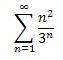# Evaluate infinite sum

• ptolema

## Homework Statement

evaluate the sum:(it may help to think of this as a value of a function defined by a power series.)

## Homework Equations

as a function defined by a power series, the function is centered at 0: f(1/3)==1/3+4/9+1/3+16/81+...+n2/3n

## The Attempt at a Solution

i'm not entirely clear on how to actually evaluate the value of an infinite series. i could definitely use a few pointers

hi ptolema!(have a sigma: ∑)

(not sure about the power series, but … )

have you tried ∑ (n+1)2/3n - ∑ n2/3n ?ok so how about considering a taylor series about zero as a start
$$f(a) = \sum_{n=0} f^n(a) x^n$$

$$0 + 1.\frac{1}{3} + 2^2.\frac{1}{3^2} +3^2.\frac{1}{3^3} +...+ n^2 (\frac{1}{3^n})$$
$$\sum_{n=1}^{\infty} \frac{x^n}{3^n} = \frac{x}{3-x}$$ for |x| < 3.
$$\sum_{n=1}^{\infty} \frac{n^2}{3^n} = \sum_{n=0}^{\infty} \frac{(n+1)^2}{3^{n+1}} = \frac{1}{3}\sum_{n=0}^{\infty} \frac{n^2 + 2n +1}{3^n} =...$$